Core Java - Interview Questions and Answers for 'Lambda expression' | Search Interview Question - javasearch.buggybread.com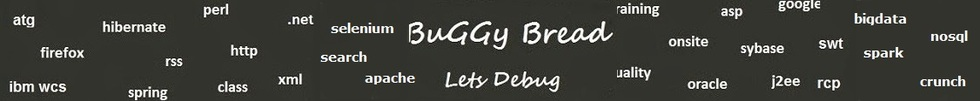# Search Interview QuestionsMore than 3000 questions in repository.There are more than 900 unanswered questions.Have a video suggestion.
Click Correct / Improve and please let us know.
Label / Company      Label / Company / TextSubmit Question

Core Java - Interview Questions and Answers for 'Lambda expression' - 12 question(s) found - Order By Newest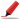Frequently asked at Manhattan Associates ( Based on 2 feedback )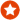Q1. What is a Lambda Expression ? What's its use ? Core Java
Ans. Its an anonymous method without any declaration.

Lambda Expression are useful to write shorthand Code and hence saves the effort of writing lengthy Code.

It promotes Developer productivity, Better Readable and Reliable code.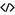Sample Code for lambdaHelp us improve. Please let us know the company, where you were asked this question :LikeDiscussCorrect / Improvejava   java8   lambda expression   architecture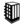Asked in 58 Companies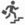expert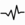frequent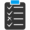Try 1 Question(s) TestNot frequently asked as it was introduced with Java 8.Q2. Difference between final and effectively final ? Why is effectively final even required ? Core Java
Ans. Final variable means a variable that has been declared final and hence cannot be de referenced after initialization. Effective final means a variable that has not been declared final but haven't been reassigned the value after initialization.

First is the regulation that restricts the reassignment and will raise a compilation error if we try to do so. Second is the outcome without the restriction.

Effective Final is the eventual treatment of the variable that is required for many features. For eq - Java 8 requires that local variables referenced from a lambda expression must be final or effectively final.It means all local referenced from lambda expressions must be such that their value shouldn't be changed after initialization whether declared final or not.Help us improve. Please let us know the company, where you were asked this question :LikeDiscussCorrect / Improvejava   java8   java 8   final   effective final   final vs effective final   lambda expressionsexpertTry 2 Question(s) TestQ3. Difference between Predicate, Supplier and Consumer ? Core Java
Ans. Predicate represents an anonymous function that accepts one argument and produces a result.

Supplier represents an anonymous function that accepts no argument and produces a result.

Consumer represents an anonymous function that accepts an argument and produces no result.Sample Code for PredicateSample Code for SupplierSample Code for ConsumerHelp us improve. Please let us know the company, where you were asked this question :LikeDiscussCorrect / Improvejava   java8   predicate   consumer   supplier   lambda expression   predicate vs consumer vs supplierexpertQ4. Write a Program that gets a set of numbers , filters out the non prime numbers , calculate the factorial of each prime number and then finds the average of all factorials using Lambda expressions
Ans. public class BuggyBread1 {

public static void main(String args[]) {

// Declare and Initialize the Collection
Set<Integer> intSet = new HashSet<Integer>();

double averageOfNonPrimeFactorials = intSet.stream().filter(p->checkIfPrime(p)).collect(Collectors.averagingInt(p->calculateFactorial(p)));

System.out.println(averageOfNonPrimeFactorials );
}

static private boolean checkIfPrime(int num){
for(int count=2;count < num;count++){
if(num % count == 0){
return false;
}
}
return true;
}

static private int calculateFactorial(int num){
int factorial = 1;
for(int count=num;count > 0;count--){
factorial = factorial * count;
}
return factorial;
}
}Help us improve. Please let us know the company, where you were asked this question :LikeDiscussCorrect / Improvejava 8  lambda expressions  lambda  filter  Collectors  factorial  prime numberQ5. What does the following lambda expression means ?helloJava8 ( x-> x%2 )
Ans. helloJava8 receives an Integer as argument and then returns the modulus of that Integer.Help us improve. Please let us know the company, where you were asked this question :LikeDiscussCorrect / Improvejava   java8   lambda expressionRarely asked as it was introduced with Java 8.Q6. Difference between DoubleSummaryStatistics , IntSummaryStatistics and LongSummaryStatistics ? Core Java
Ans. They all does the same task i.e to compute statistical information on the stream of data. They differ by the way they store the statistical information as they expect a different data type of the values being used.

IntSummaryStatistics and LongSummaryStatistics expect non floating point values and hence stores the statistical information like min,max and sum as non floating values ( int or long ) whereas DoubleSummaryStatistics stores these information as floating value.Help us improve. Please let us know the company, where you were asked this question :LikeDiscussCorrect / Improvejava   java8   java 8   lambda expressions   doublesummarystatistics   intsummarystatistics   longsummarystatistics   summarystatisticsRarely asked as it was introduced with Java 8.Q7. What is the use of :: operator wef from Java 8 ? Core Java
Ans. We can refer to a function using this operator like System.out.println(intList.stream().reduce(Math::max).get());Help us improve. Please let us know the company, where you were asked this question :LikeDiscussCorrect / Improve:: operator   lambda expression  java 8Q8. What is a method reference in Java ? Core Java
Ans. Introduced with java 8 , Method References help us to point to methods by their name.

A method references is described using :: symbolHelp us improve. Please let us know the company, where you were asked this question :LikeDiscussCorrect / Improvemethod reference  java 8  lambda expressionsQ9. Lets say we have a set "set1" with objects of Class A, Class has a non static string called element1, Write a code ( using lambda expression ) to see if there is any object in the set with element1 = "Hello". Core Java
Ans. set1.stream().filter(p->p.element1.equals("Hello")).findAny().isPreent();

or

set1.stream().map(A::getElement1).anyMatch("Hello"::equals)Help us improve. Please let us know the company, where you were asked this question :LikeDiscussCorrect / Improvelambda expressions  java 8  java8  java 8 filter  findAny()Q10. Have you used Java 8 Lambda expressions ? Core JavaThis question was recently asked at 'One Click Retail'.This question is still unanswered. Can you please provide an answer.Help us improve. Please let us know the company, where you were asked this question :LikeDiscussCorrect / Improvejava 8  lambda expressionsAsked in 1 CompaniesfrequentQ11. What are the advantages and disadvantages of Lambda Expression ? Core JavaThis question was recently asked at 'Number 8'.This question is still unanswered. Can you please provide an answer.Help us improve. Please let us know the company, where you were asked this question :LikeDiscussCorrect / Improvejava8  java 8  java lambda expressionsAsked in 1 CompaniesQ12. What is arrow function in Typescript ? TypeScriptThis question is still unanswered. Can you please provide an answer.Help us improve. Please let us know the company, where you were asked this question :LikeDiscussCorrect / Improvearraow functions in typescript   lambda expression vs arrow function

## Help us and Others Improve. Please let us know the questions asked in any of your previous interview.

Any input from you will be highly appreciated and It will unlock the application for 10 more requests.

 Company Name: Questions Asked: